Foreign Exchange Risk Foreign Exchange Risk Foreign exchange

• Slides: 46Foreign Exchange RiskForeign Exchange Risk • Foreign exchange risk is the risk that the value of an asset or liability will change because of a change in exchange rates. • Because these international obligations span time, foreign exchange risk can arise.Sources of Risk • Transaction Exposure: The risk that the domestic cost or proceeds of a transaction may change. • Translation Exposure: The risk that the translation of value of foreign-currency-denominated assets is affect by exchange rate changes. • Economic Exposure: The risk that exchange rate changes may affect the present value of future income streams.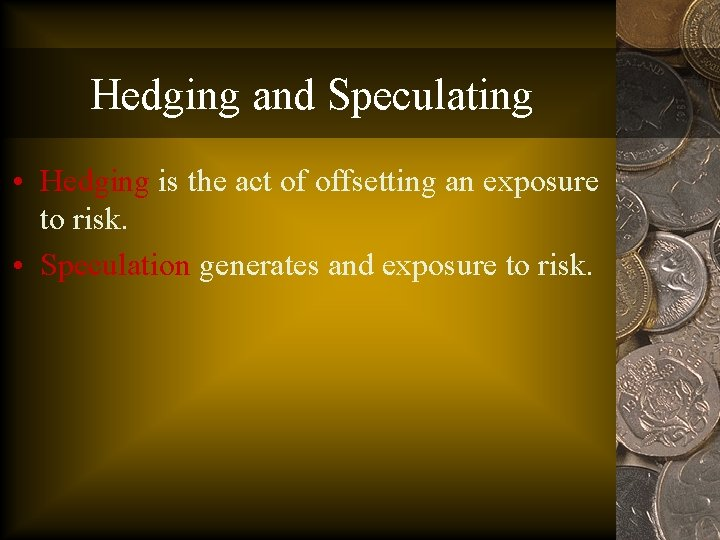Hedging and Speculating • Hedging is the act of offsetting an exposure to risk. • Speculation generates and exposure to risk.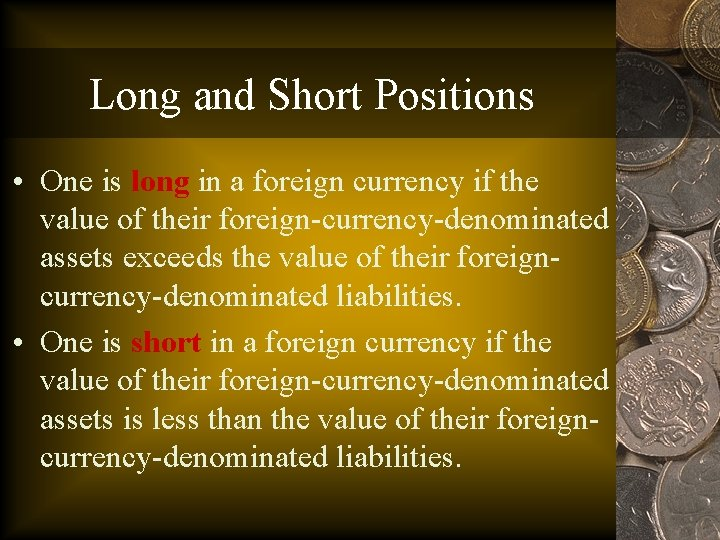Long and Short Positions • One is long in a foreign currency if the value of their foreign-currency-denominated assets exceeds the value of their foreigncurrency-denominated liabilities. • One is short in a foreign currency if the value of their foreign-currency-denominated assets is less than the value of their foreigncurrency-denominated liabilities.Hedging • There a number of instruments that can be used to hedge foreign exchange risk. • Chapter 4 deals with the forward markets, while Chapter 5 introduces foreign exchange futures, options, and swaps.Forward Exchange Contracts The market for future delivery of a currency.Forward Market • The forward market is the market for the future delivery of a currency. • Typical maturity is 1, 3, 6, 9 and 12 months. • The forward rate is determined by market participants’ expectations of the future spot value of the currency which, in turn, depends on other economic variables. • Hence, the forward market may provide some information about future spot price movements.Forward Premium • The difference between the spot and forward rates is expressed as the standard (or annualized) forward premium or discount. • The standard premium is calculated as the difference between the two rates as a percent of the spot rate, and then annualized (simple basis).Example • For example, suppose the spot rate is 1. 6035 (\$/£) and the 3 -month forward rate is 1. 6050. • The forward premium on the pound is: [(1. 6050 -1. 6035)/1. 6035]*(12/3)*100 = 0. 37%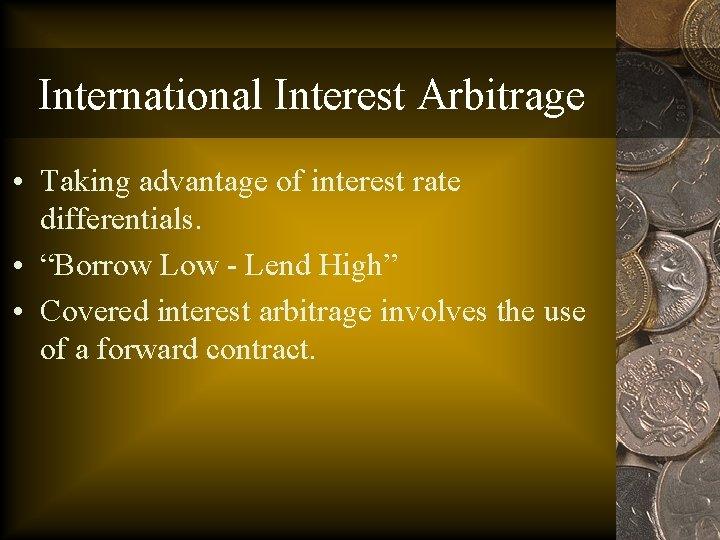International Interest Arbitrage • Taking advantage of interest rate differentials. • “Borrow Low - Lend High” • Covered interest arbitrage involves the use of a forward contract.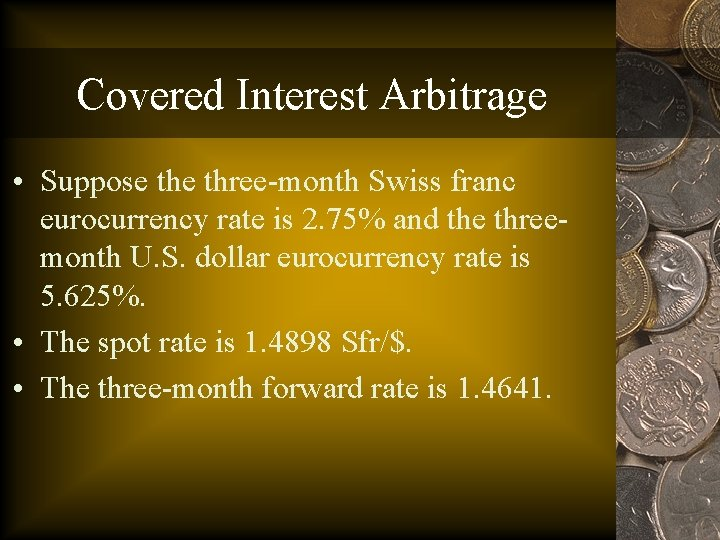Covered Interest Arbitrage • Suppose three-month Swiss franc eurocurrency rate is 2. 75% and the threemonth U. S. dollar eurocurrency rate is 5. 625%. • The spot rate is 1. 4898 Sfr/\$. • The three-month forward rate is 1. 4641.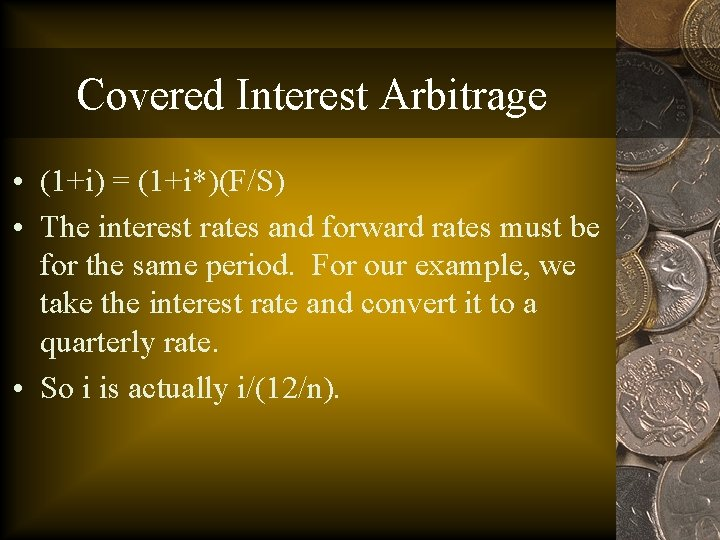Covered Interest Arbitrage • (1+i) = (1+i*)(F/S) • The interest rates and forward rates must be for the same period. For our example, we take the interest rate and convert it to a quarterly rate. • So i is actually i/(12/n).Example Continued • Substitute the values into the condition: [1+(0. 0275/4)]=[1+(0. 05625/4)](1. 4641/1. 4898) (1. 006875)=(1. 0140625)(0. 98275) 1. 006875 > 0. 99656 • Would want to borrow the dollar and lend the franc.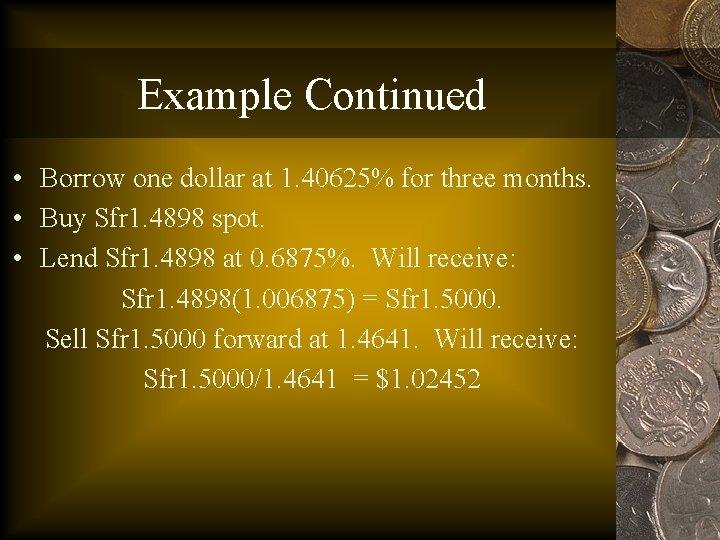Example Continued • Borrow one dollar at 1. 40625% for three months. • Buy Sfr 1. 4898 spot. • Lend Sfr 1. 4898 at 0. 6875%. Will receive: Sfr 1. 4898(1. 006875) = Sfr 1. 5000. Sell Sfr 1. 5000 forward at 1. 4641. Will receive: Sfr 1. 5000/1. 4641 = \$1. 02452Example Continued • Repay dollar loan at a cost of; \$1(1. 0140625) = \$1. 0140625. • Profit of \$1. 02452 - \$1. 0140625 = \$0. 0105, or 1. 05%.Covered Interest Parity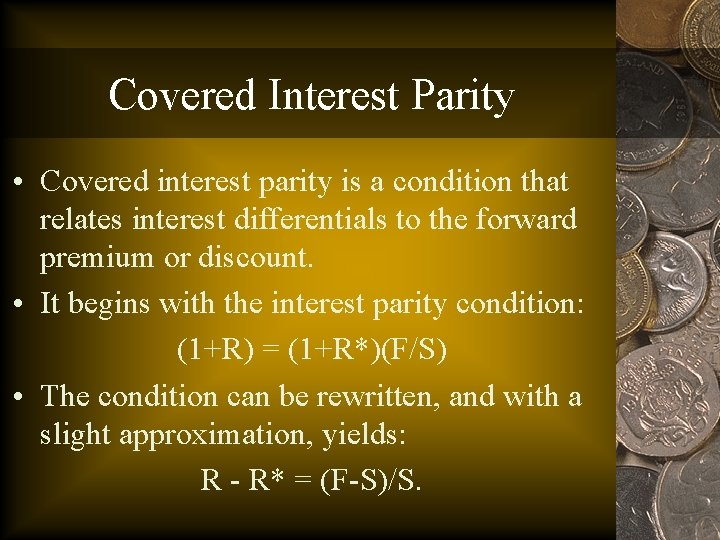Covered Interest Parity • Covered interest parity is a condition that relates interest differentials to the forward premium or discount. • It begins with the interest parity condition: (1+R) = (1+R*)(F/S) • The condition can be rewritten, and with a slight approximation, yields: R - R* = (F-S)/S.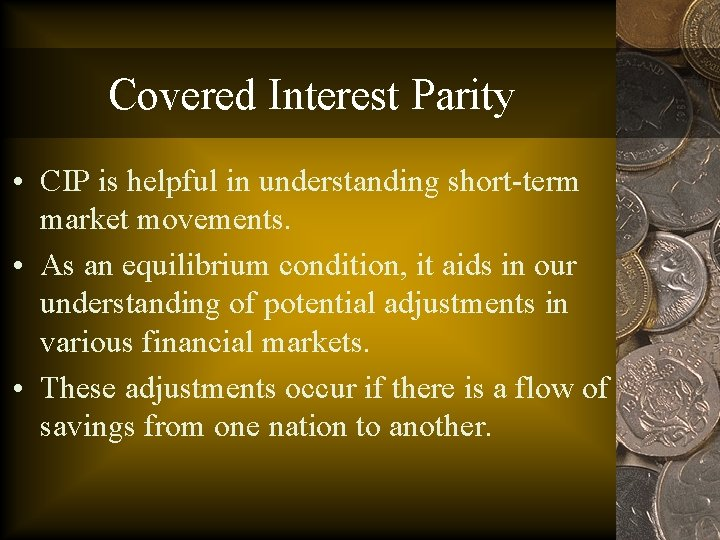Covered Interest Parity • CIP is helpful in understanding short-term market movements. • As an equilibrium condition, it aids in our understanding of potential adjustments in various financial markets. • These adjustments occur if there is a flow of savings from one nation to another.Example • Suppose the 90 -day U. S. interest rate is 5. 5% while the U. K. rate on a similar instrument is 5. 0% (both expressed as annual rates). • The current spot rate is 1. 4546 (\$/£) and the threemonth forward rate is 1. 4900. • To use the uncovered interest parity condition, we must convert the interest rates to quarterly values: (0. 055)/(12/3) = 0. 01375 and (0. 05)/(12/3) = 0. 0125.Example • Now substitute the values into the CIP condition: 0. 01375 - 0. 0125 = (1. 4900 -1. 4546)/1. 4546, 0. 00125 < 0. 0243. • Though the interest rate on the U. S. instrument is higher than that on the U. K. instrument, the difference is outweighed by the depreciation (forward discount) of the dollar over the time interval.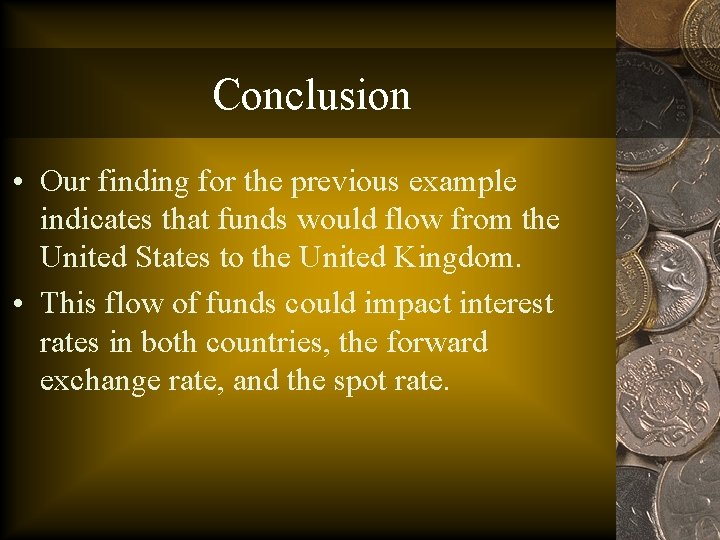Conclusion • Our finding for the previous example indicates that funds would flow from the United States to the United Kingdom. • This flow of funds could impact interest rates in both countries, the forward exchange rate, and the spot rate.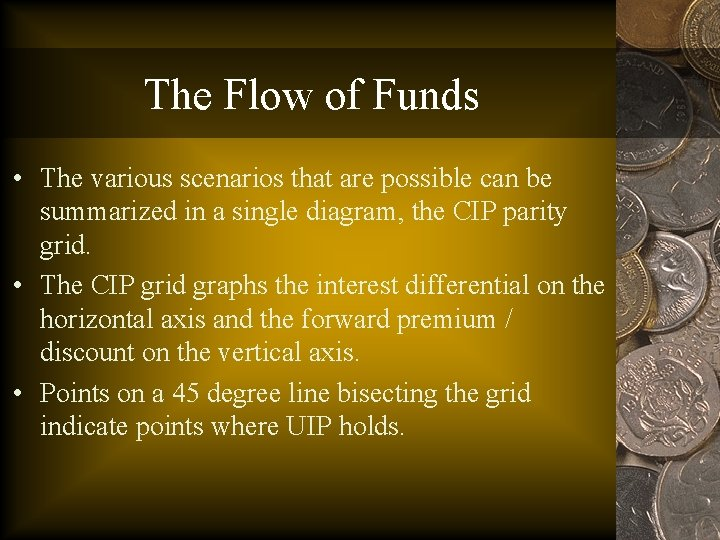The Flow of Funds • The various scenarios that are possible can be summarized in a single diagram, the CIP parity grid. • The CIP grid graphs the interest differential on the horizontal axis and the forward premium / discount on the vertical axis. • Points on a 45 degree line bisecting the grid indicate points where UIP holds.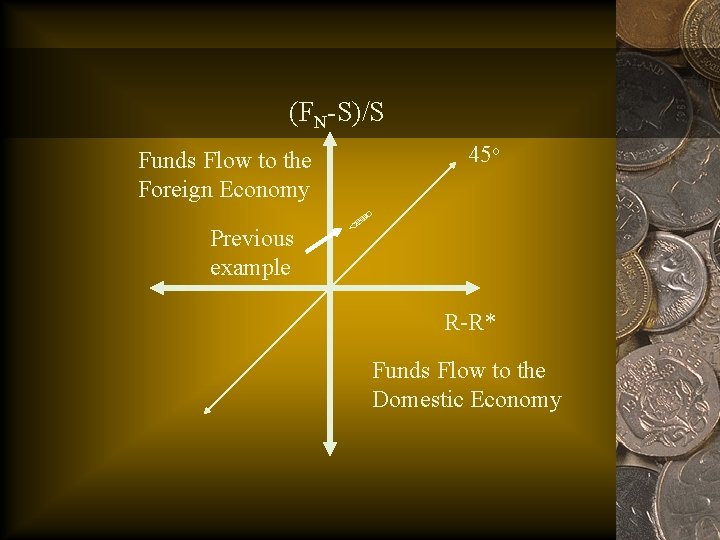(FN-S)/S 45 o Funds Flow to the Foreign Economy Previous example R-R* Funds Flow to the Domestic EconomyThe Flow of Funds • The flow of funds from the United States to the United Kingdom potentially can cause changes in the spot rate, the forward rate, and interest rates in both countries. • The following slides illustrate the diagrams for each.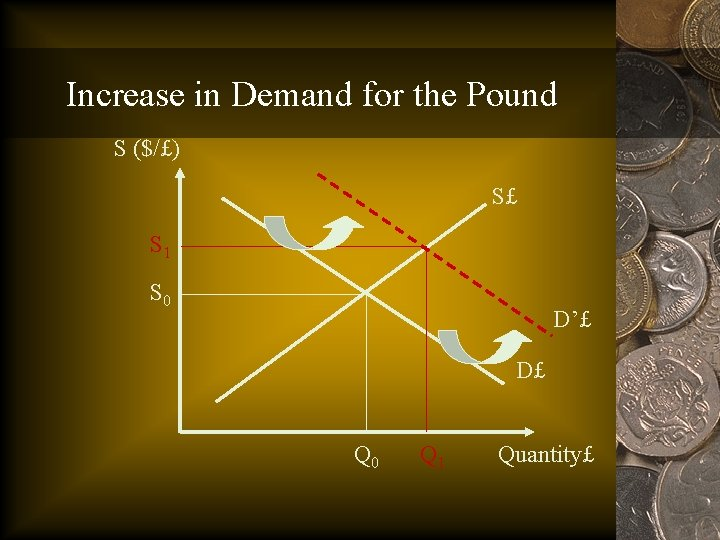Increase in Demand for the Pound S (\$/£) S£ S 1 S 0 D’£ D£ Q 0 Q 1 Quantity£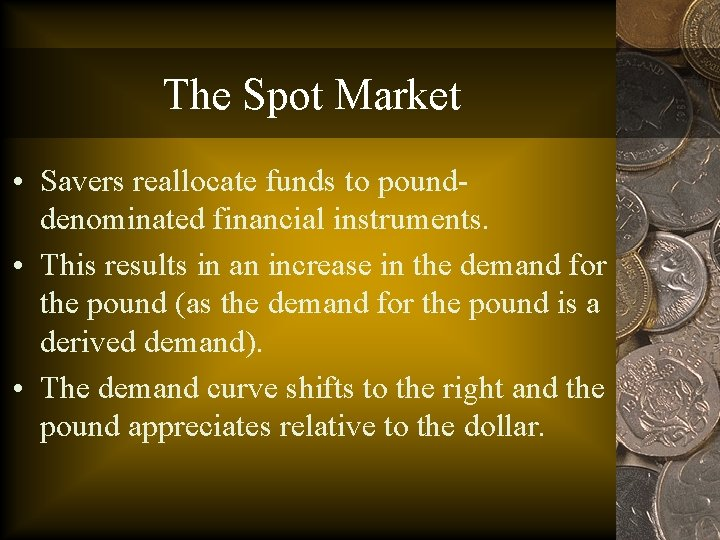The Spot Market • Savers reallocate funds to pounddenominated financial instruments. • This results in an increase in the demand for the pound (as the demand for the pound is a derived demand). • The demand curve shifts to the right and the pound appreciates relative to the dollar.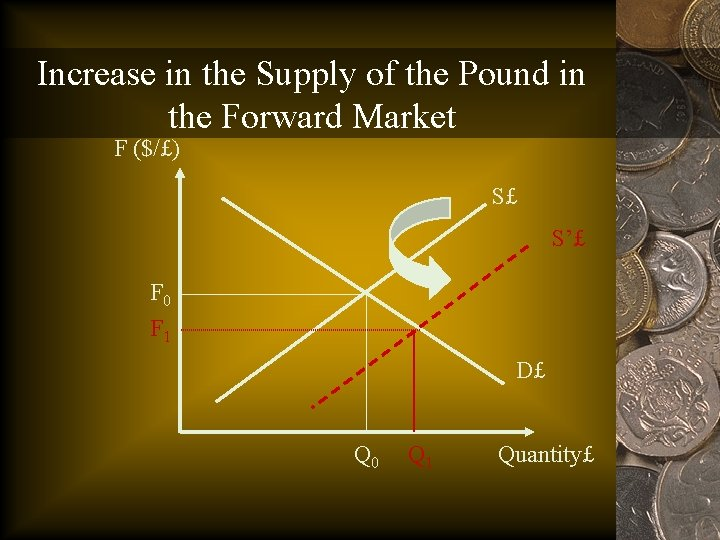Increase in the Supply of the Pound in the Forward Market F (\$/£) S£ S’£ F 0 F 1 D£ Q 0 Q 1 Quantity£The Forward Market • Because saver are now long the pound, to cover their position they must sell the pound forward. • This causes an increase in the supply of the pound in the forward market, and the value of the pound in the forward market declines.Increase in the Supply of Loanable Funds in the U. K. R* SLF S’LF R*0 R*1 DLF Q 0 Q 1 Quantity. LFLoanable Funds in the U. K. • As funds flow into the United Kingdom, the supply of loanable funds increases. • This causes the interest rate to decline in the U. K.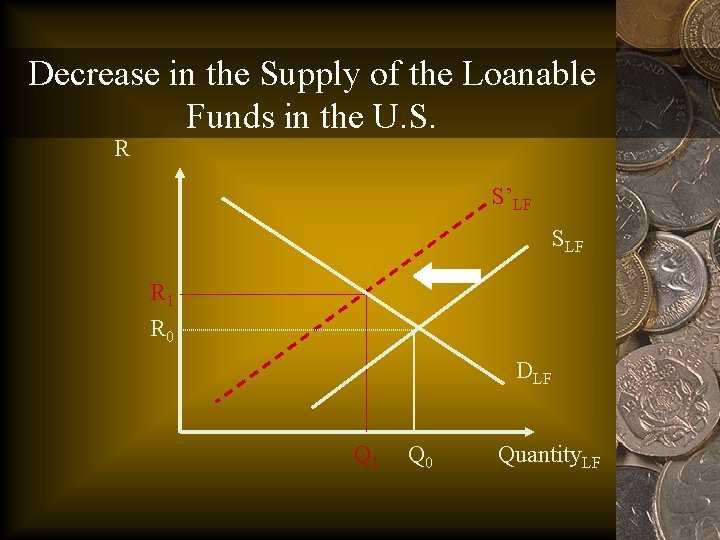Decrease in the Supply of the Loanable Funds in the U. S. R S’LF SLF R 1 R 0 DLF Q 1 Q 0 Quantity. LFLoanable Funds in the U. S. • As funds flow out of the U. S. , the supply of loanable funds in the United States declines. • This causes the interest rate to rise in the United States.Uncovered Interest Parity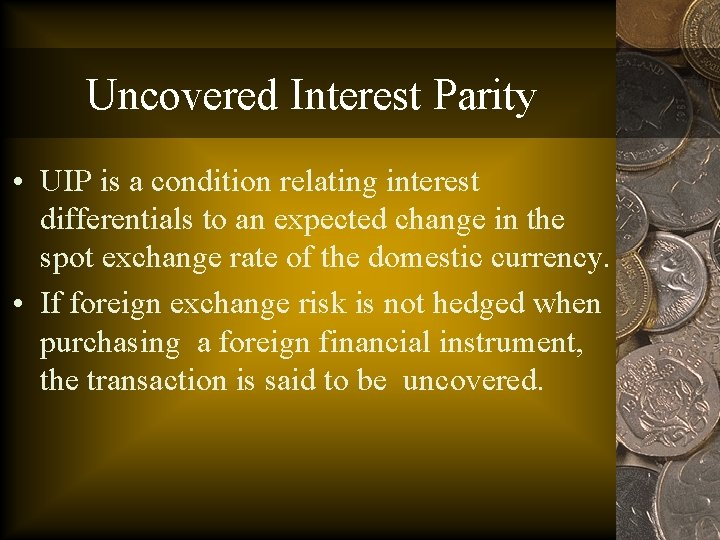Uncovered Interest Parity • UIP is a condition relating interest differentials to an expected change in the spot exchange rate of the domestic currency. • If foreign exchange risk is not hedged when purchasing a foreign financial instrument, the transaction is said to be uncovered.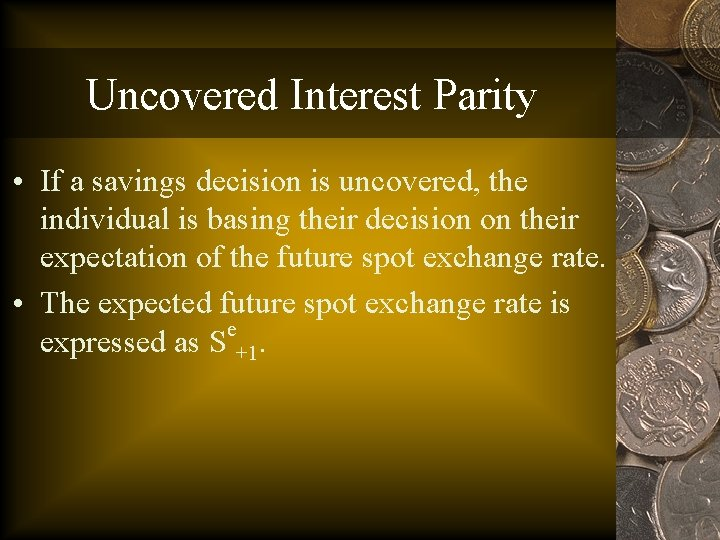Uncovered Interest Parity • If a savings decision is uncovered, the individual is basing their decision on their expectation of the future spot exchange rate. • The expected future spot exchange rate is e expressed as S +1.Uncovered Interest Parity • Using this expression for the expected future spot rate, UIP can be written as: e R – R* = (S +1 – S)/S. • In words, the right-hand-side of the UIP condition is the expected change in the spot rate over the relevant time period. • We can express the expected change in the spot rates as Se.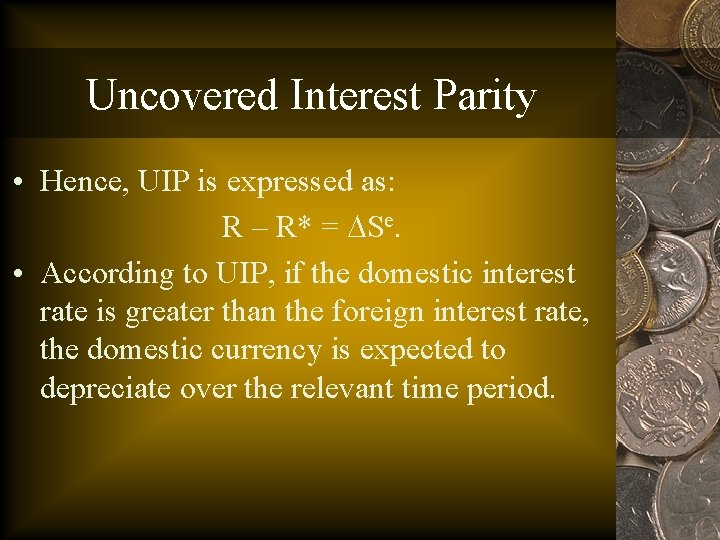Uncovered Interest Parity • Hence, UIP is expressed as: R – R* = Se. • According to UIP, if the domestic interest rate is greater than the foreign interest rate, the domestic currency is expected to depreciate over the relevant time period.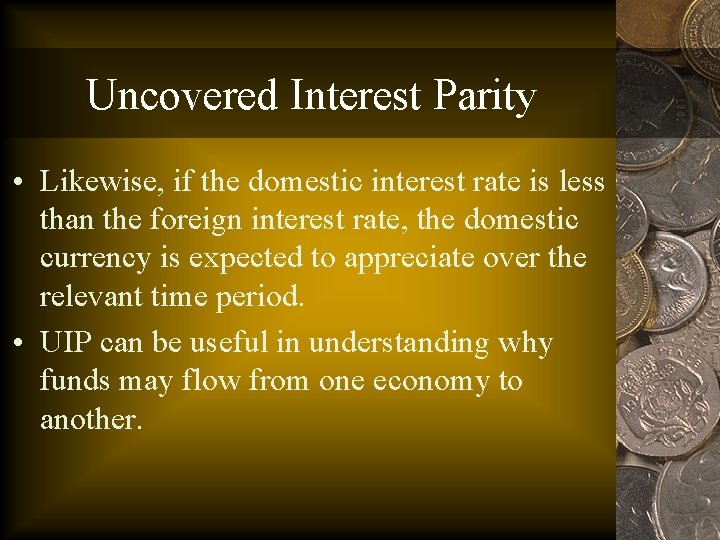Uncovered Interest Parity • Likewise, if the domestic interest rate is less than the foreign interest rate, the domestic currency is expected to appreciate over the relevant time period. • UIP can be useful in understanding why funds may flow from one economy to another.UIP as an Equilibrium Condition • Consider the following situation: R – R* > Se, with both sides positive. The interest differential in favor of the domestic financial instrument exceeds the expected depreciation of the domestic currency. In this case, and ignoring transaction costs, the saver would be induced to reallocate their funds and we would expect funds to flow to the domestic economy.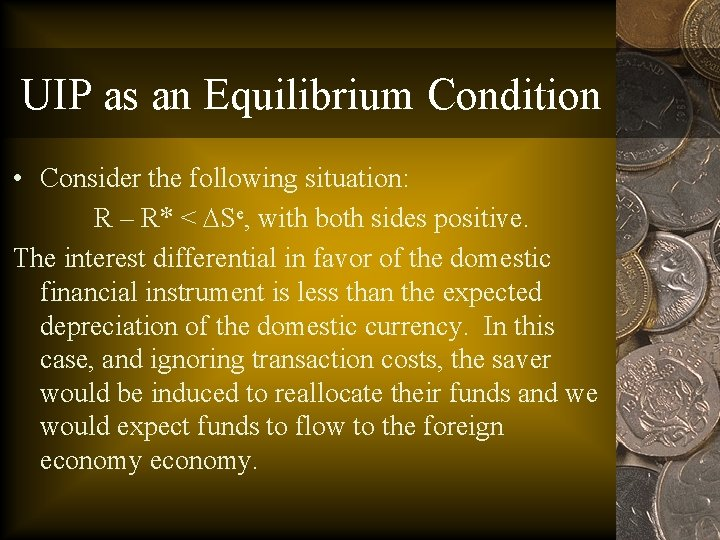UIP as an Equilibrium Condition • Consider the following situation: R – R* < Se, with both sides positive. The interest differential in favor of the domestic financial instrument is less than the expected depreciation of the domestic currency. In this case, and ignoring transaction costs, the saver would be induced to reallocate their funds and we would expect funds to flow to the foreign economy.UIP as an Equilibrium Condition • The various scenarios that are possible can be summarized in a single diagram, the UIP parity grid. • The UIP grid graphs the interest differential on the horizontal axis and the expected change in the spot exchange rate on the vertical axis. • Points on a 45 degree line bisecting the grid indicate points where UIP holds.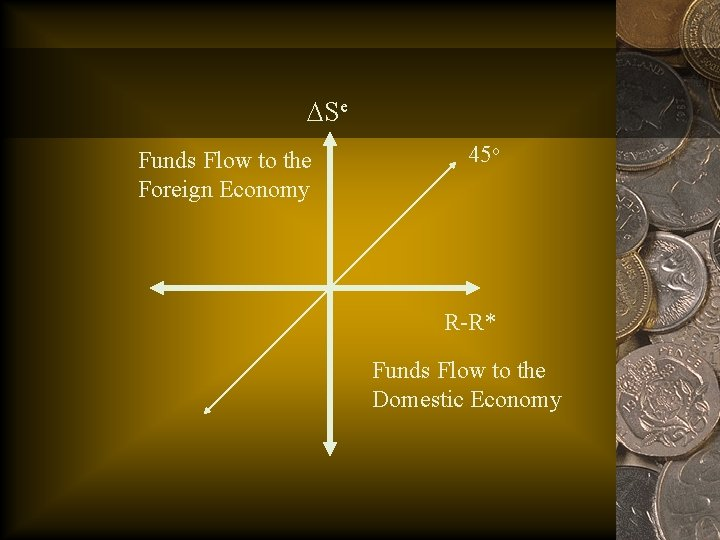Se Funds Flow to the Foreign Economy 45 o R-R* Funds Flow to the Domestic EconomyDeviations from UIP • To examine the performance of UIP, we can rewrite the basic interest arbitrage equation: (1+R) = (1+R*)(Se+1/S), As Se+1 = S(1+R)/(1+R*). • Se+1 is then the UIP spot rate, which can be compared to the actual spot rate that prevailed at time period +1.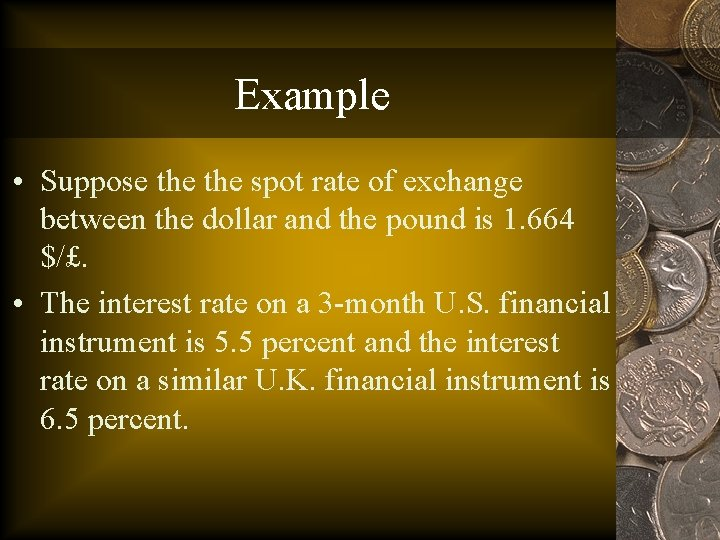Example • Suppose the spot rate of exchange between the dollar and the pound is 1. 664 \$/£. • The interest rate on a 3 -month U. S. financial instrument is 5. 5 percent and the interest rate on a similar U. K. financial instrument is 6. 5 percent.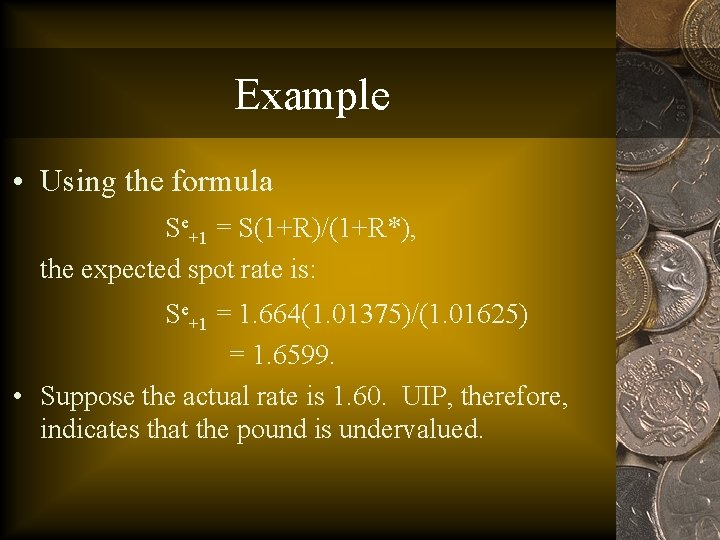Example • Using the formula Se+1 = S(1+R)/(1+R*), the expected spot rate is: Se+1 = 1. 664(1. 01375)/(1. 01625) = 1. 6599. • Suppose the actual rate is 1. 60. UIP, therefore, indicates that the pound is undervalued.